Скачать презентацию Kinetics Effects in Multiple Intra-beam Scattering IBS P

f30ab096c43a18df1fc35eb378db0e1c.ppt

• Количество слайдов: 39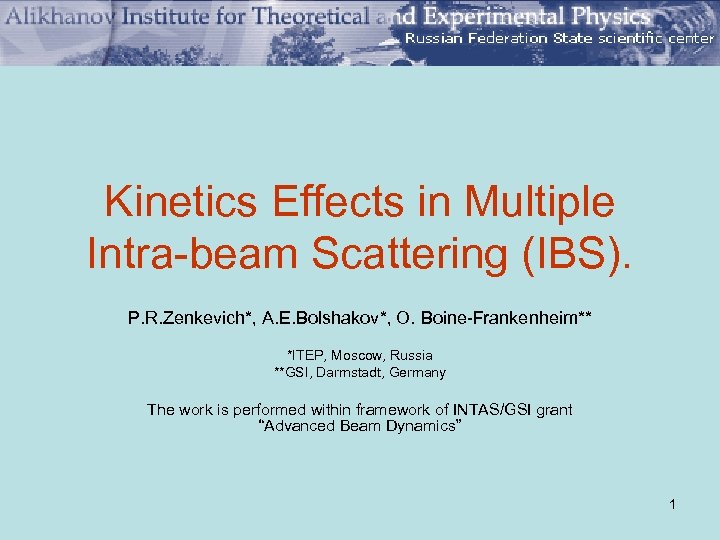Kinetics Effects in Multiple Intra-beam Scattering (IBS). P. R. Zenkevich*, A. E. Bolshakov*, O. Boine-Frankenheim** *ITEP, Moscow, Russia **GSI, Darmstadt, Germany The work is performed within framework of INTAS/GSI grant “Advanced Beam Dynamics” 1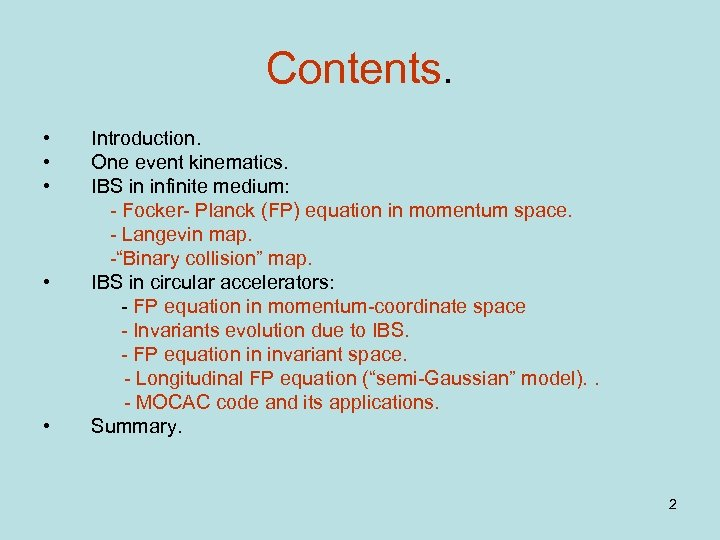Contents. • • • Introduction. One event kinematics. IBS in infinite medium: - Focker- Planck (FP) equation in momentum space. - Langevin map. -“Binary collision” map. IBS in circular accelerators: - FP equation in momentum-coordinate space - Invariants evolution due to IBS. - FP equation in invariant space. - Longitudinal FP equation (“semi-Gaussian” model). . - MOCAC code and its applications. Summary. 2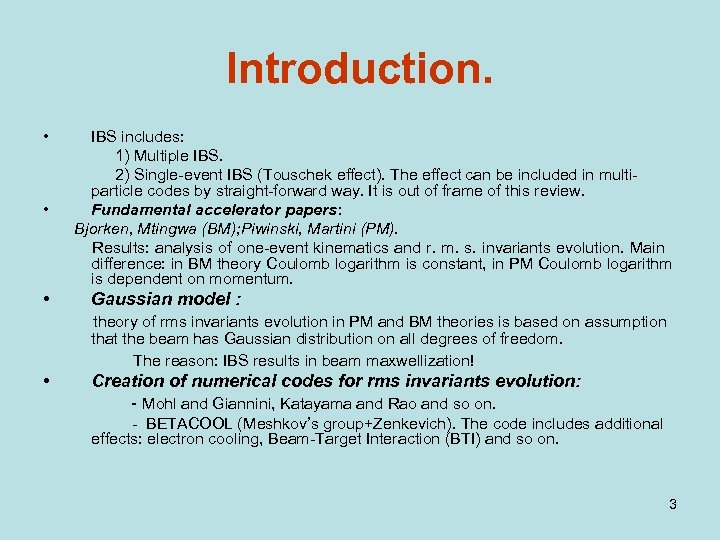Introduction. • • • IBS includes: 1) Multiple IBS. 2) Single-event IBS (Touschek effect). The effect can be included in multiparticle codes by straight-forward way. It is out of frame of this review. Fundamental accelerator papers: Bjorken, Mtingwa (BM); Piwinski, Martini (PM). Results: analysis of one-event kinematics and r. m. s. invariants evolution. Main difference: in BM theory Coulomb logarithm is constant, in PM Coulomb logarithm is dependent on momentum. Gaussian model : theory of rms invariants evolution in PM and BM theories is based on assumption that the beam has Gaussian distribution on all degrees of freedom. The reason: IBS results in beam maxwellization! • Creation of numerical codes for rms invariants evolution: - Mohl and Giannini, Katayama and Rao and so on. - BETACOOL (Meshkov’s group+Zenkevich). The code includes additional effects: electron cooling, Beam-Target Interaction (BTI) and so on. 3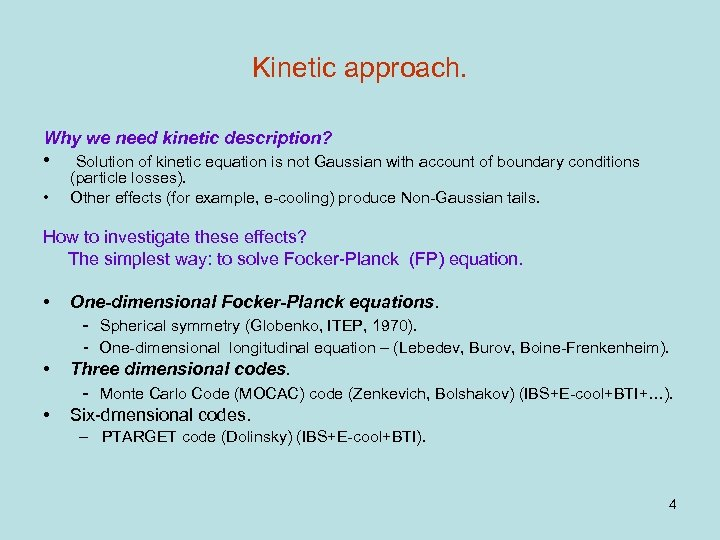Kinetic approach. Why we need kinetic description? • • Solution of kinetic equation is not Gaussian with account of boundary conditions (particle losses). Other effects (for example, e-cooling) produce Non-Gaussian tails. How to investigate these effects? The simplest way: to solve Focker-Planck (FP) equation. • One-dimensional Focker-Planck equations. - Spherical symmetry (Globenko, ITEP, 1970). - One-dimensional longitudinal equation – (Lebedev, Burov, Boine-Frenkenheim). • Three dimensional codes. • Monte Carlo Code (MOCAC) code (Zenkevich, Bolshakov) (IBS+E-cool+BTI+…). Six-dmensional codes. – PTARGET code (Dolinsky) (IBS+E-cool+BTI). 4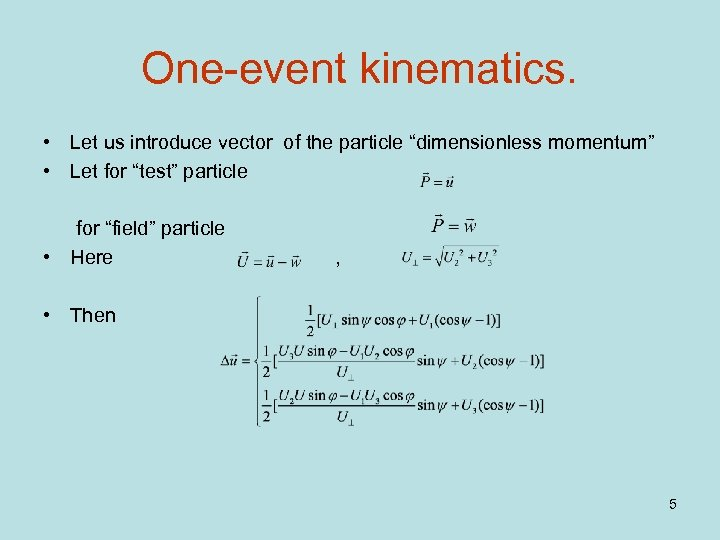One-event kinematics. • Let us introduce vector of the particle “dimensionless momentum” • Let for “test” particle for “field” particle • Here , • Then 5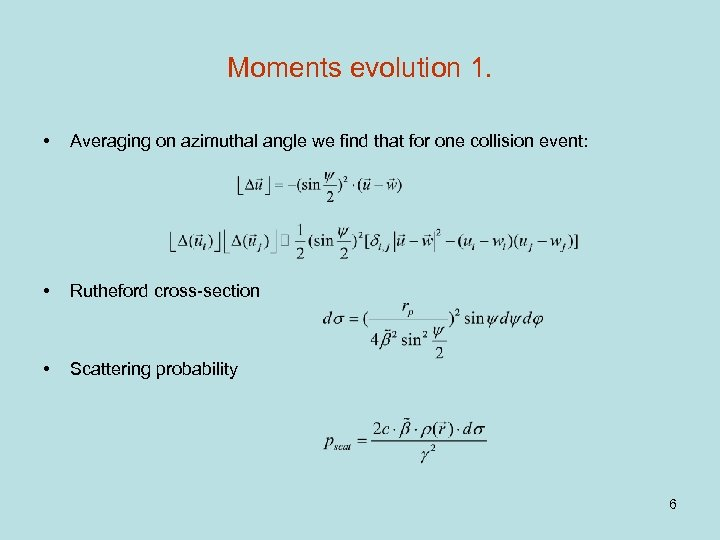Moments evolution 1. • Averaging on azimuthal angle we find that for one collision event: • Rutheford cross-section • Scattering probability 6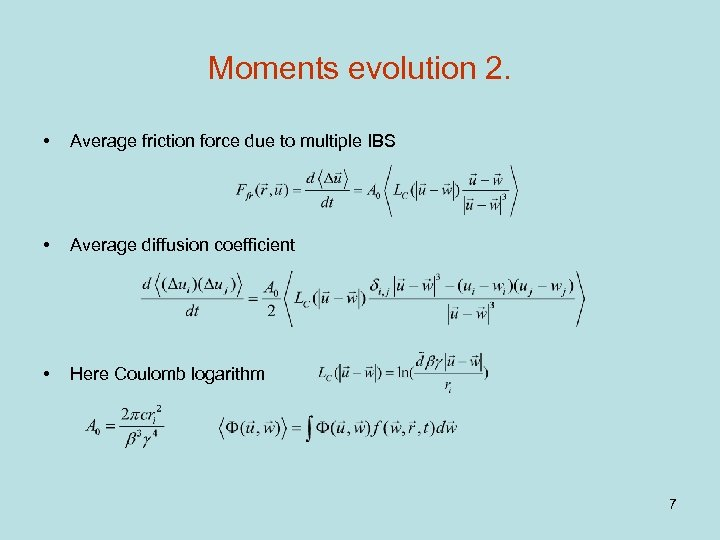Moments evolution 2. • Average friction force due to multiple IBS • Average diffusion coefficient • Here Coulomb logarithm 7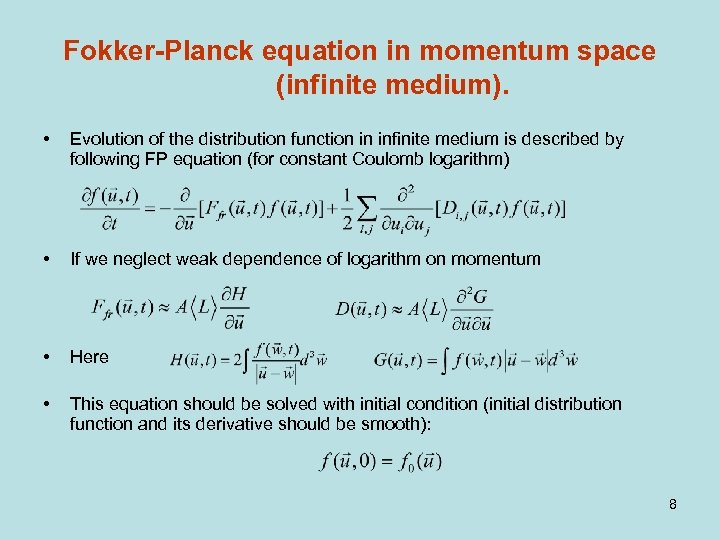Fokker-Planck equation in momentum space (infinite medium). • Evolution of the distribution function in infinite medium is described by following FP equation (for constant Coulomb logarithm) • If we neglect weak dependence of logarithm on momentum • Here • This equation should be solved with initial condition (initial distribution function and its derivative should be smooth): 8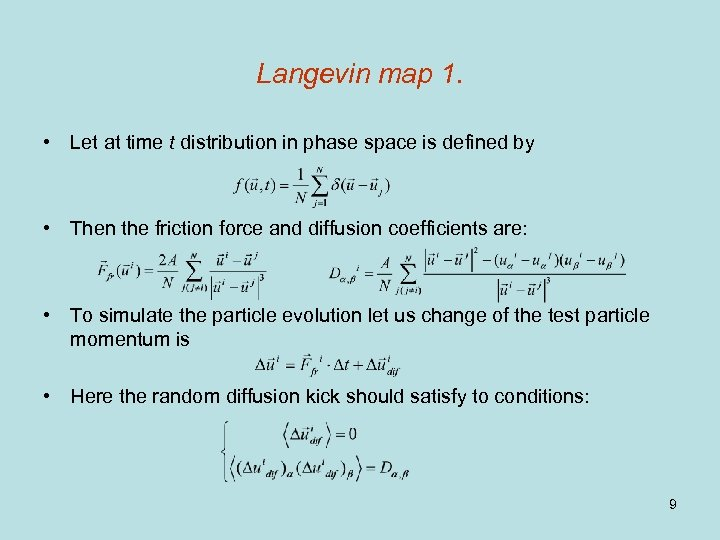Langevin map 1. • Let at time t distribution in phase space is defined by • Then the friction force and diffusion coefficients are: • To simulate the particle evolution let us change of the test particle momentum is • Here the random diffusion kick should satisfy to conditions: 9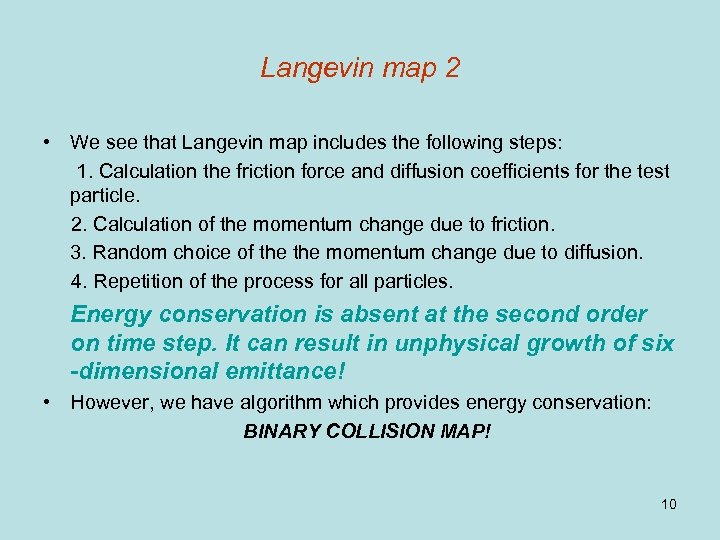Langevin map 2 • We see that Langevin map includes the following steps: 1. Calculation the friction force and diffusion coefficients for the test particle. 2. Calculation of the momentum change due to friction. 3. Random choice of the momentum change due to diffusion. 4. Repetition of the process for all particles. Energy conservation is absent at the second order on time step. It can result in unphysical growth of six -dimensional emittance! • However, we have algorithm which provides energy conservation: BINARY COLLISION MAP! 10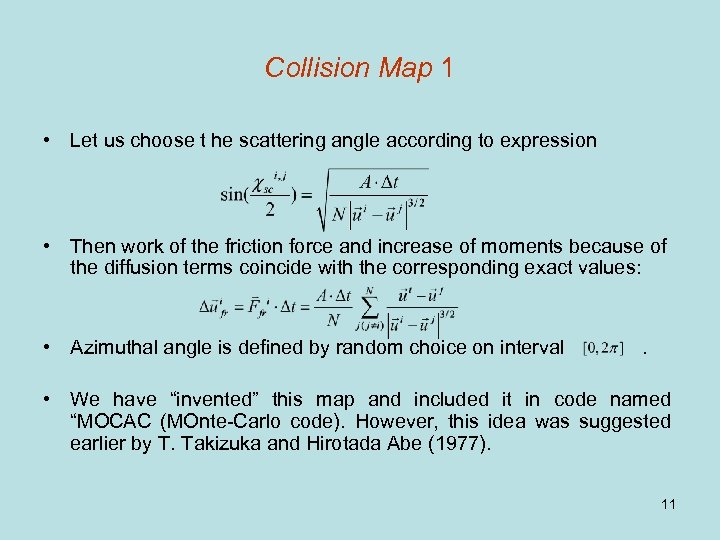Collision Map 1 • Let us choose t he scattering angle according to expression • Then work of the friction force and increase of moments because of the diffusion terms coincide with the corresponding exact values: • Azimuthal angle is defined by random choice on interval . • We have “invented” this map and included it in code named “MOCAC (MOnte-Carlo code). However, this idea was suggested earlier by T. Takizuka and Hirotada Abe (1977). 11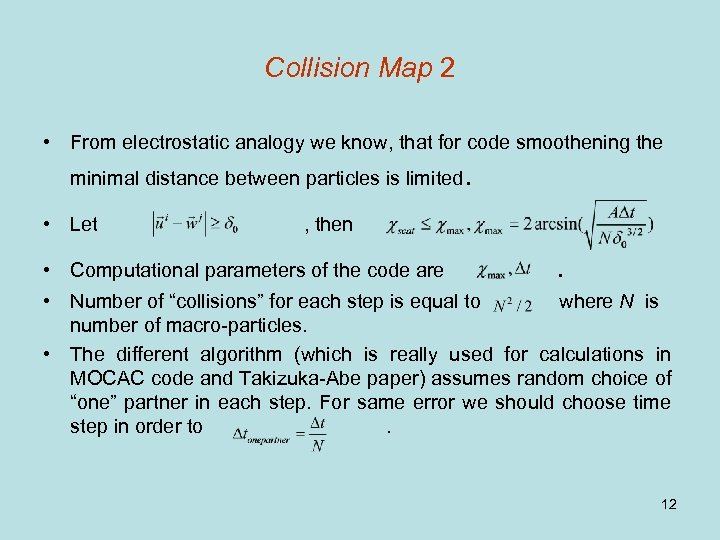Collision Map 2 • From electrostatic analogy we know, that for code smoothening the minimal distance between particles is limited • Let . , then • Computational parameters of the code are . • Number of “collisions” for each step is equal to where N is number of macro-particles. • The different algorithm (which is really used for calculations in MOCAC code and Takizuka-Abe paper) assumes random choice of “one” partner in each step. For same error we should choose time step in order to. 12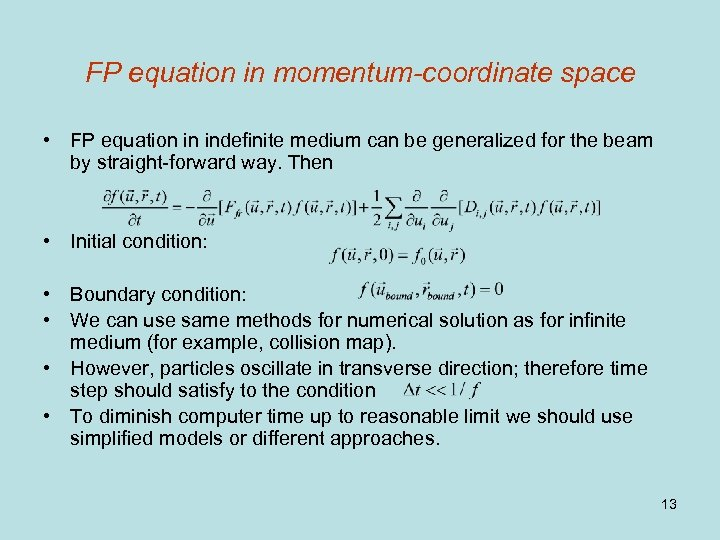FP equation in momentum-coordinate space • FP equation in indefinite medium can be generalized for the beam by straight-forward way. Then • Initial condition: • Boundary condition: • We can use same methods for numerical solution as for infinite medium (for example, collision map). • However, particles oscillate in transverse direction; therefore time step should satisfy to the condition • To diminish computer time up to reasonable limit we should use simplified models or different approaches. 13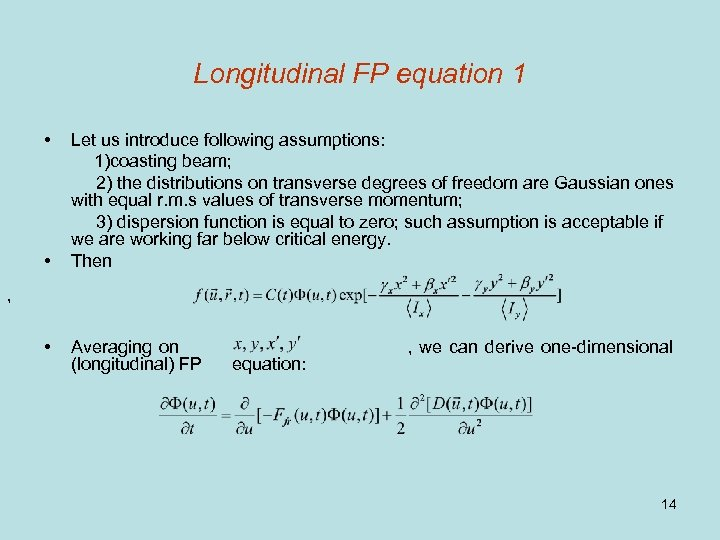Longitudinal FP equation 1 • • Let us introduce following assumptions: 1)coasting beam; 2) the distributions on transverse degrees of freedom are Gaussian ones with equal r. m. s values of transverse momentum; 3) dispersion function is equal to zero; such assumption is acceptable if we are working far below critical energy. Then , • Averaging on (longitudinal) FP equation: , we can derive one-dimensional 14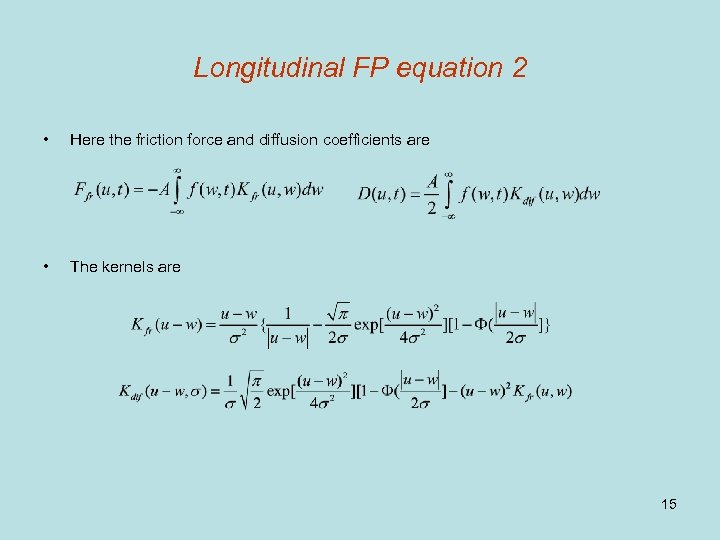Longitudinal FP equation 2 • Here the friction force and diffusion coefficients are • The kernels are 15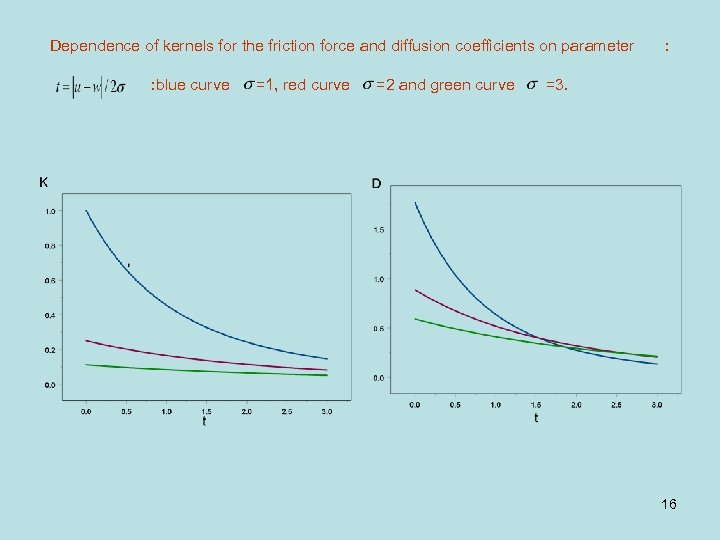Dependence of kernels for the friction force and diffusion coefficients on parameter : blue curve =1, red curve =2 and green curve : =3. 16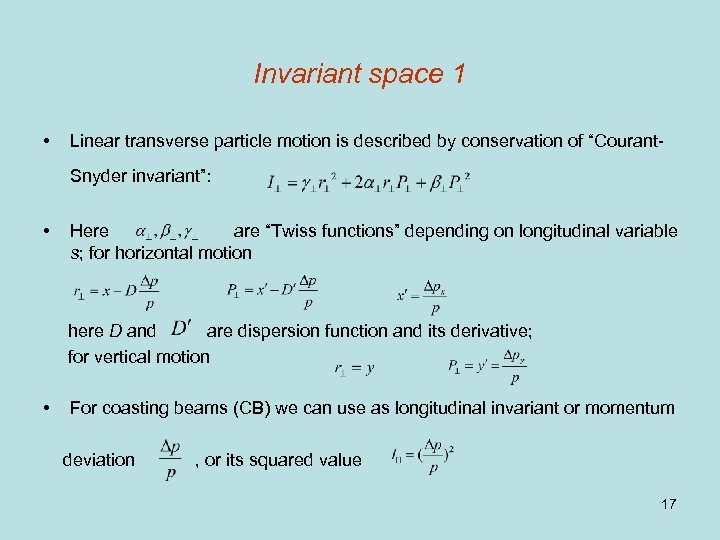Invariant space 1 • Linear transverse particle motion is described by conservation of “Courant. Snyder invariant”: • Here are “Twiss functions” depending on longitudinal variable s; for horizontal motion here D and are dispersion function and its derivative; for vertical motion • For coasting beams (CB) we can use as longitudinal invariant or momentum deviation , or its squared value 17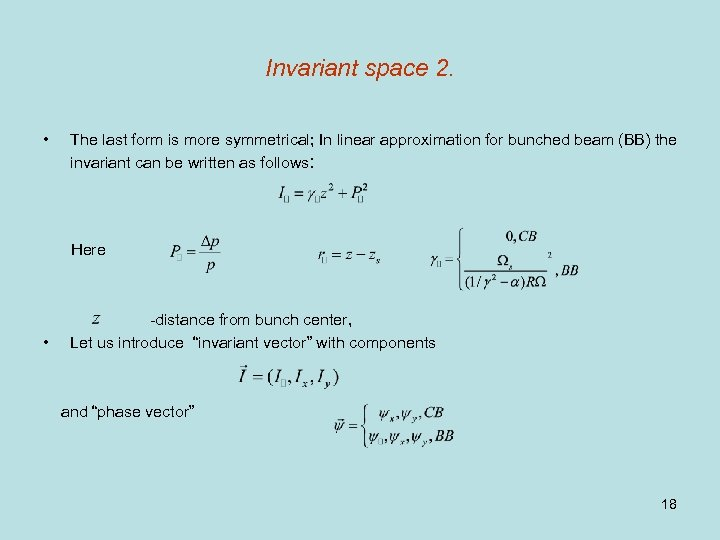Invariant space 2. • The last form is more symmetrical; In linear approximation for bunched beam (BB) the invariant can be written as follows: Here • -distance from bunch center, Let us introduce “invariant vector” with components and “phase vector” 18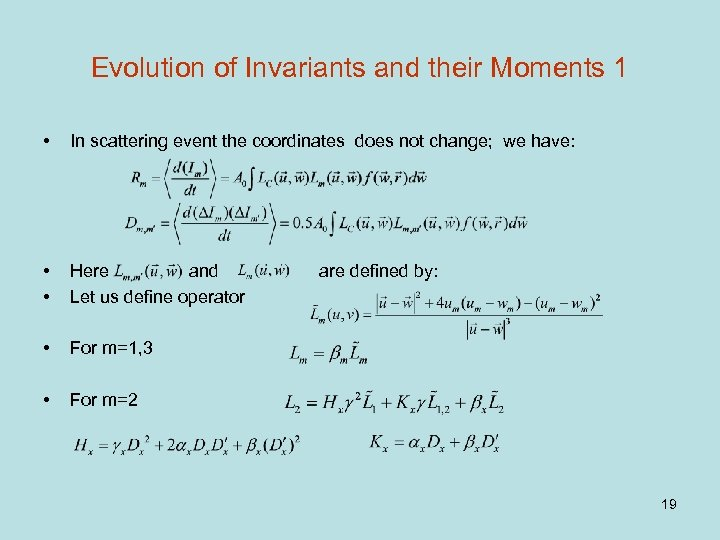Evolution of Invariants and their Moments 1 • In scattering event the coordinates does not change; we have: • • Here and Let us define operator • For m=1, 3 • For m=2 are defined by: 19Evolution of Invariants and their Moments 2 • For the second order moments of the invariants with m=1, 3 we find: • For the second order of invariant with m=2 • Here the coefficients • For the invariant with m=1, 2 20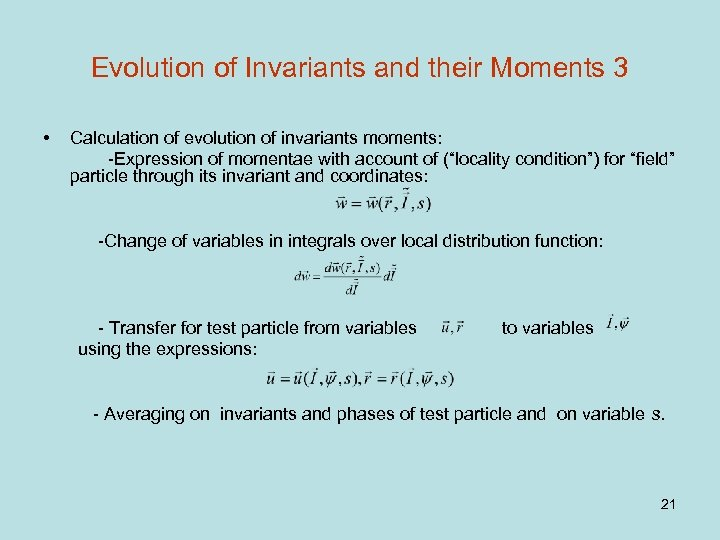Evolution of Invariants and their Moments 3 • Calculation of evolution of invariants moments: -Expression of momentae with account of (“locality condition”) for “field” particle through its invariant and coordinates: -Change of variables in integrals over local distribution function: - Transfer for test particle from variables using the expressions: to variables - Averaging on invariants and phases of test particle and on variable s. 21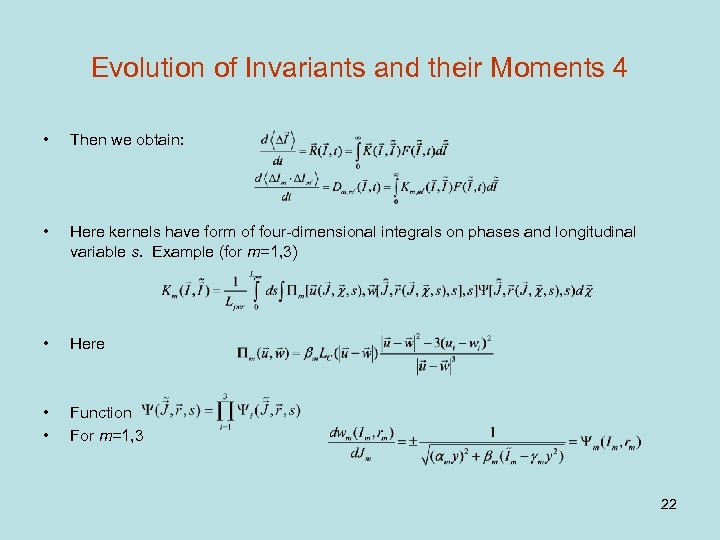Evolution of Invariants and their Moments 4 • Then we obtain: • Here kernels have form of four-dimensional integrals on phases and longitudinal variable s. Example (for m=1, 3) • Here • • Function For m=1, 3 22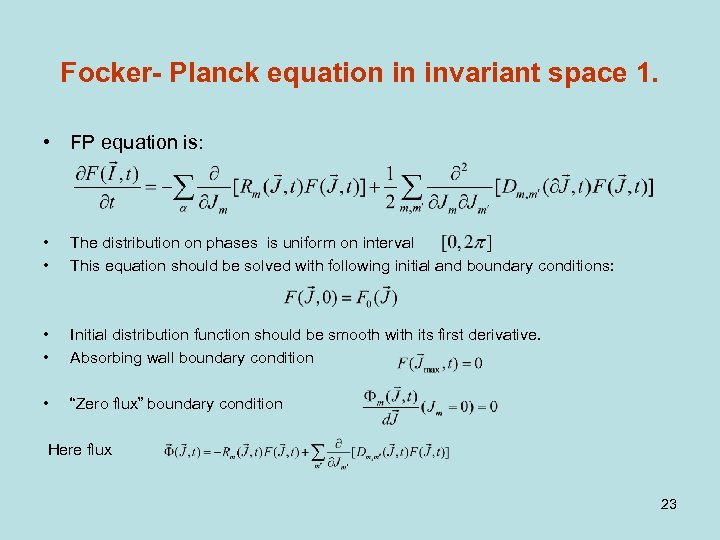Focker- Planck equation in invariant space 1. • FP equation is: • • The distribution on phases is uniform on interval This equation should be solved with following initial and boundary conditions: • • Initial distribution function should be smooth with its first derivative. Absorbing wall boundary condition • “Zero flux” boundary condition Here flux 2324Application of Langevin method for solution of FP equation in invariant space 1. • If we know the kernels we can use for numerical solution “Langevin method”. Let • Then • Change of invariant: • Here change of the invariant due to “friction” is defined by: 25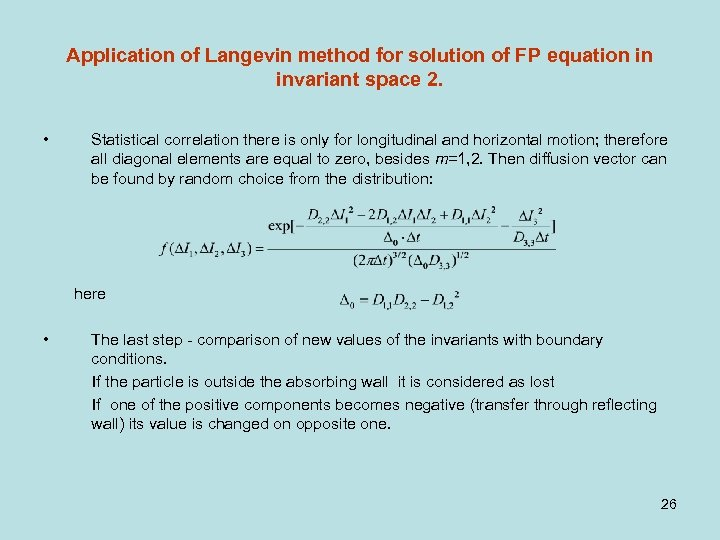Application of Langevin method for solution of FP equation in invariant space 2. • Statistical correlation there is only for longitudinal and horizontal motion; therefore all diagonal elements are equal to zero, besides m=1, 2. Then diffusion vector can be found by random choice from the distribution: here • The last step - comparison of new values of the invariants with boundary conditions. If the particle is outside the absorbing wall it is considered as lost If one of the positive components becomes negative (transfer through reflecting wall) its value is changed on opposite one. 26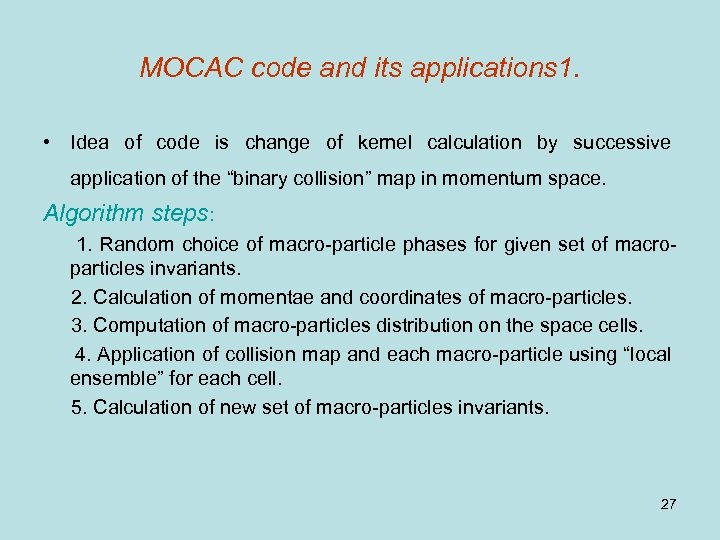MOCAC code and its applications 1. • Idea of code is change of kernel calculation by successive application of the “binary collision” map in momentum space. Algorithm steps: 1. Random choice of macro-particle phases for given set of macroparticles invariants. 2. Calculation of momentae and coordinates of macro-particles. 3. Computation of macro-particles distribution on the space cells. 4. Application of collision map and each macro-particle using “local ensemble” for each cell. 5. Calculation of new set of macro-particles invariants. 27MOCAC code and its applications 2. • This operation can be considered as “collective map” in invariant space; each particle is “test” and “field” particle simultaneously. • The map is repeated through the time interval • If the magnetic lattice is non-uniform, it is presented as set of discrete points corresponding different longitudinal coordinates. Each point is characterized by its set of Twiss parameters and dispersion function. The points are distributed uniformly on the lattice period. • The map is made through each time interval in new point of period (averaging on the lattice). 28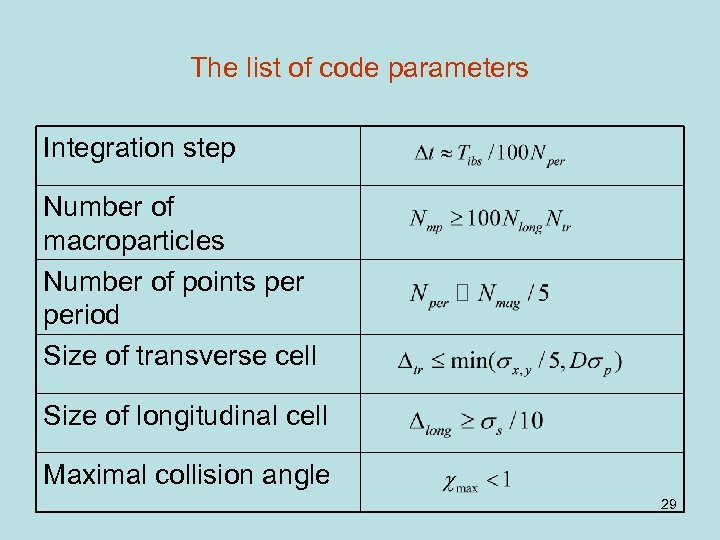The list of code parameters Integration step Number of macroparticles Number of points period Size of transverse cell Size of longitudinal cell Maximal collision angle 29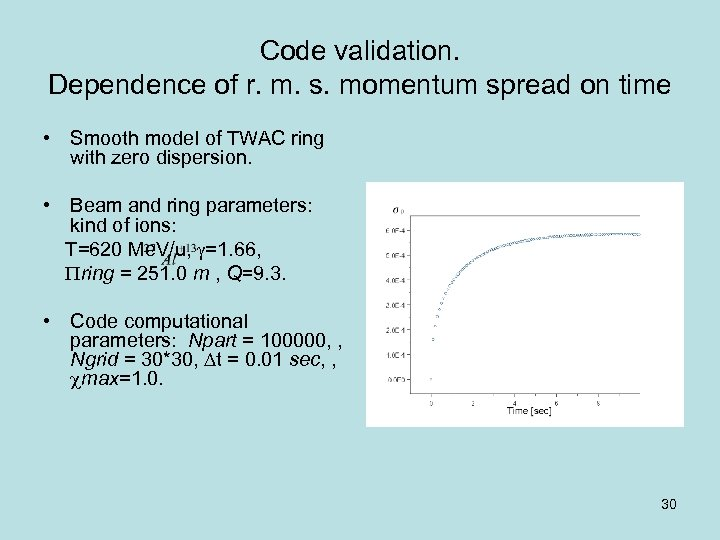Code validation. Dependence of r. m. s. momentum spread on time • Smooth model of TWAC ring with zero dispersion. • Beam and ring parameters: kind of ions: T=620 Me. V/u, =1. 66, ring = 251. 0 m , Q=9. 3. • Code computational parameters: Npart = 100000, , Ngrid = 30*30, t = 0. 01 sec, , max=1. 0. 30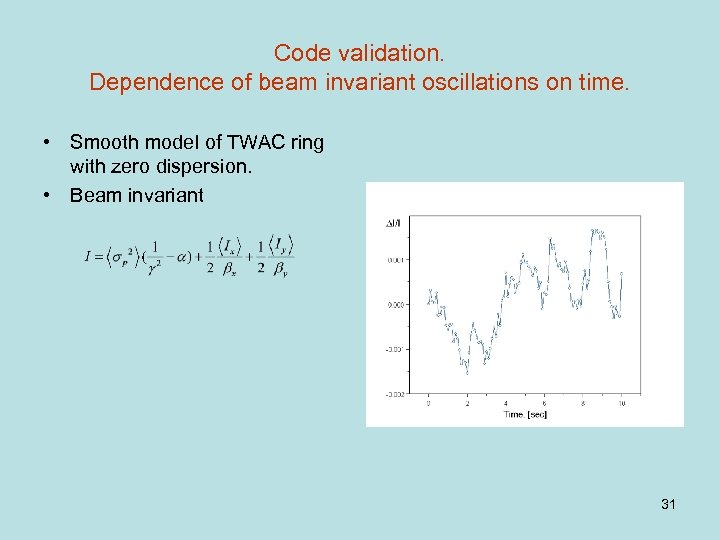Code validation. Dependence of beam invariant oscillations on time. • Smooth model of TWAC ring with zero dispersion. • Beam invariant 31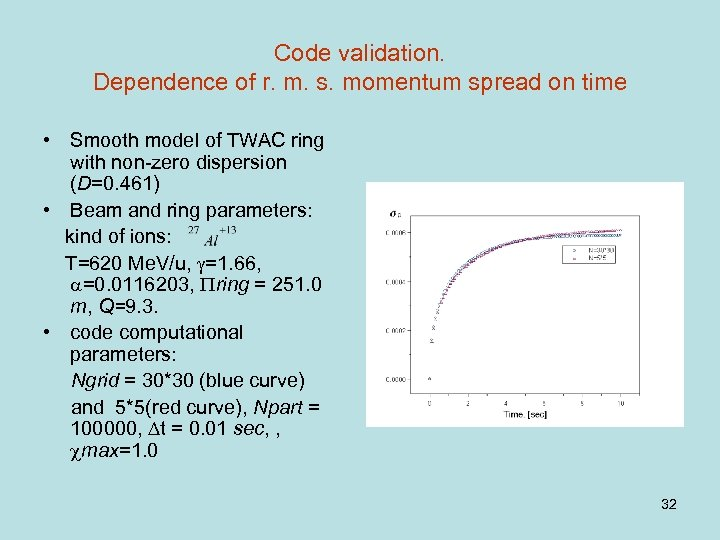Code validation. Dependence of r. m. s. momentum spread on time • Smooth model of TWAC ring with non-zero dispersion (D=0. 461) • Beam and ring parameters: kind of ions: T=620 Me. V/u, =1. 66, =0. 0116203, ring = 251. 0 m, Q=9. 3. • code computational parameters: Ngrid = 30*30 (blue curve) and 5*5(red curve), Npart = 100000, t = 0. 01 sec, , max=1. 0 32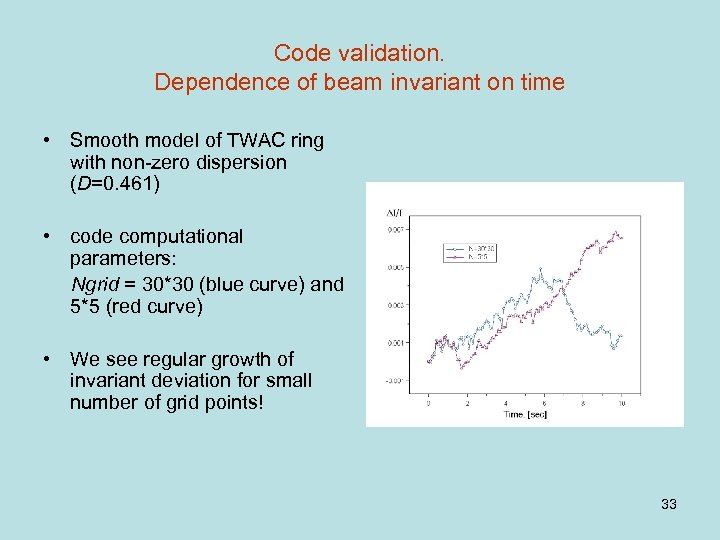Code validation. Dependence of beam invariant on time • Smooth model of TWAC ring with non-zero dispersion (D=0. 461) • code computational parameters: Ngrid = 30*30 (blue curve) and 5*5 (red curve) • We see regular growth of invariant deviation for small number of grid points! 33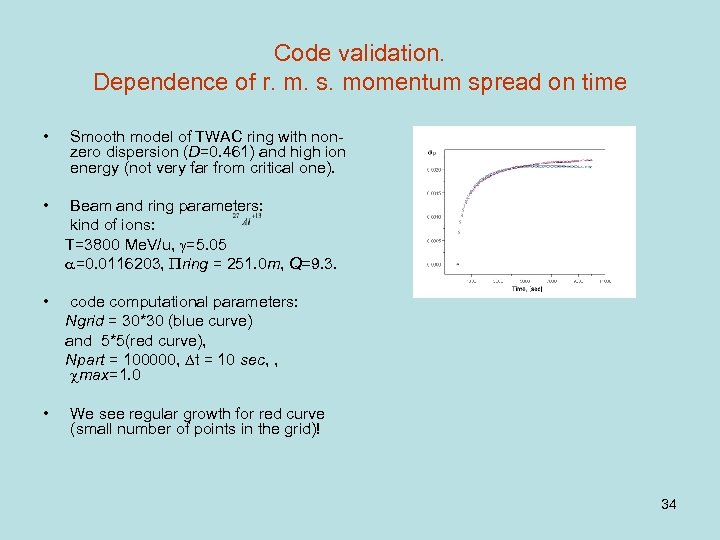Code validation. Dependence of r. m. s. momentum spread on time • Smooth model of TWAC ring with nonzero dispersion (D=0. 461) and high ion energy (not very far from critical one). • Beam and ring parameters: kind of ions: T=3800 Me. V/u, =5. 05 =0. 0116203, ring = 251. 0 m, Q=9. 3. • code computational parameters: Ngrid = 30*30 (blue curve) and 5*5(red curve), Npart = 100000, t = 10 sec, , max=1. 0 • We see regular growth for red curve (small number of points in the grid)! 34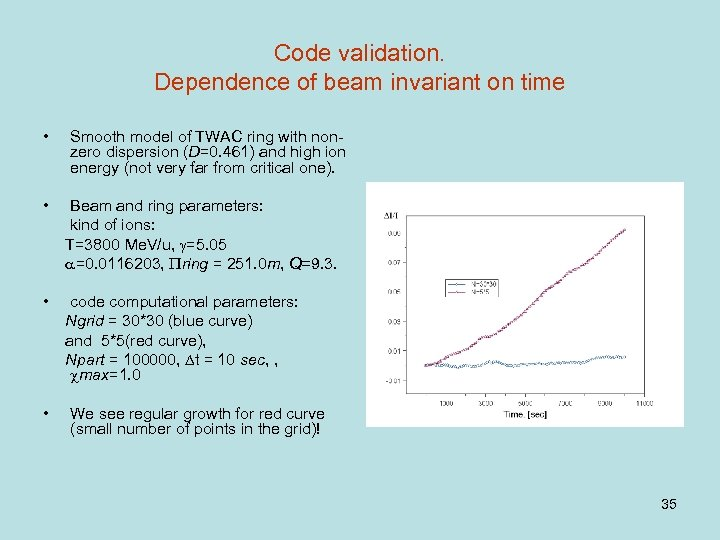Code validation. Dependence of beam invariant on time • Smooth model of TWAC ring with nonzero dispersion (D=0. 461) and high ion energy (not very far from critical one). • Beam and ring parameters: kind of ions: T=3800 Me. V/u, =5. 05 =0. 0116203, ring = 251. 0 m, Q=9. 3. • code computational parameters: Ngrid = 30*30 (blue curve) and 5*5(red curve), Npart = 100000, t = 10 sec, , max=1. 0 • We see regular growth for red curve (small number of points in the grid)! 35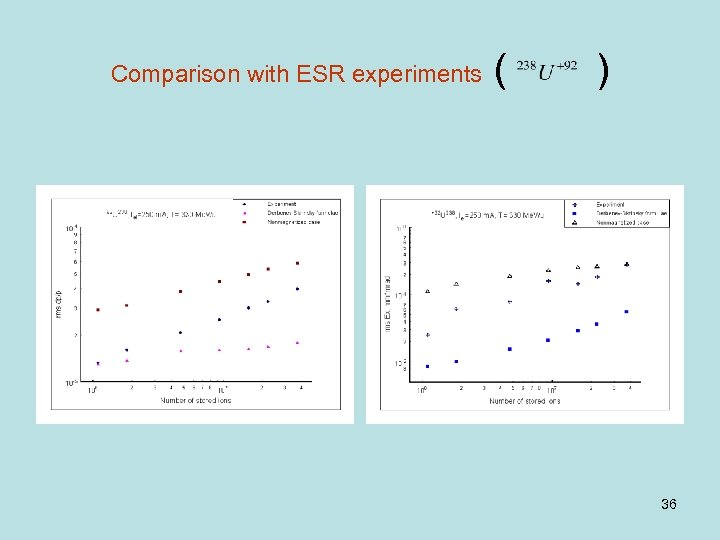Comparison with ESR experiments ( ) 36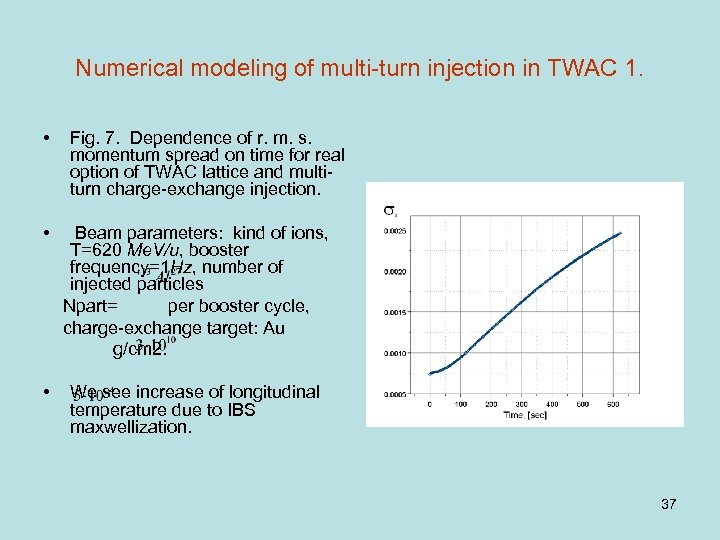Numerical modeling of multi-turn injection in TWAC 1. • Fig. 7. Dependence of r. m. s. momentum spread on time for real option of TWAC lattice and multiturn charge-exchange injection. • Beam parameters: kind of ions, T=620 Me. V/u, booster frequency=1 Hz, number of injected particles Npart= per booster cycle, charge-exchange target: Au g/cm 2. • We see increase of longitudinal temperature due to IBS maxwellization. 37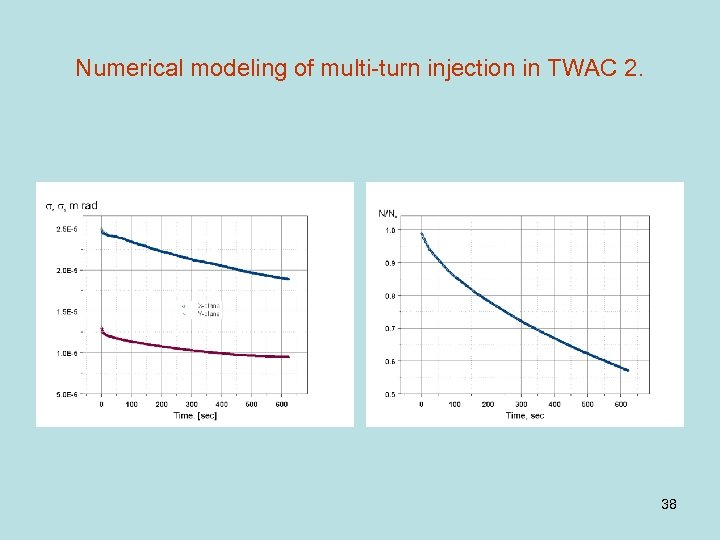Numerical modeling of multi-turn injection in TWAC 2. 38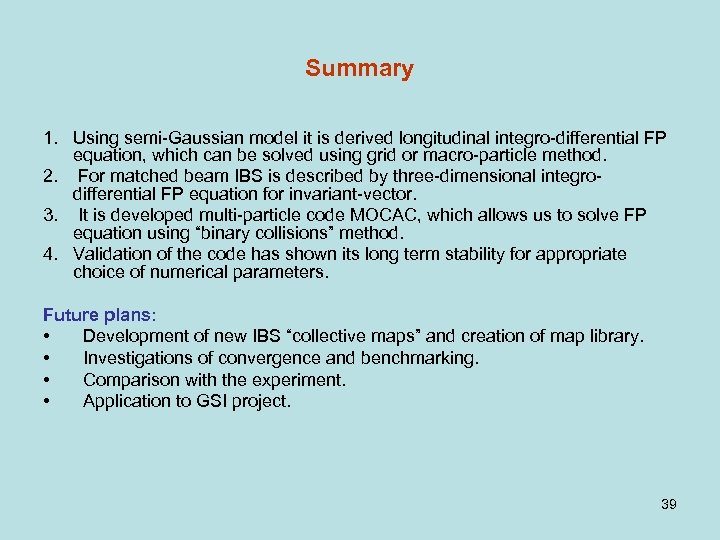Summary 1. Using semi-Gaussian model it is derived longitudinal integro-differential FP equation, which can be solved using grid or macro-particle method. 2. For matched beam IBS is described by three-dimensional integrodifferential FP equation for invariant-vector. 3. It is developed multi-particle code MOCAC, which allows us to solve FP equation using “binary collisions” method. 4. Validation of the code has shown its long term stability for appropriate choice of numerical parameters. Future plans: • Development of new IBS “collective maps” and creation of map library. • Investigations of convergence and benchmarking. • Comparison with the experiment. • Application to GSI project. 39